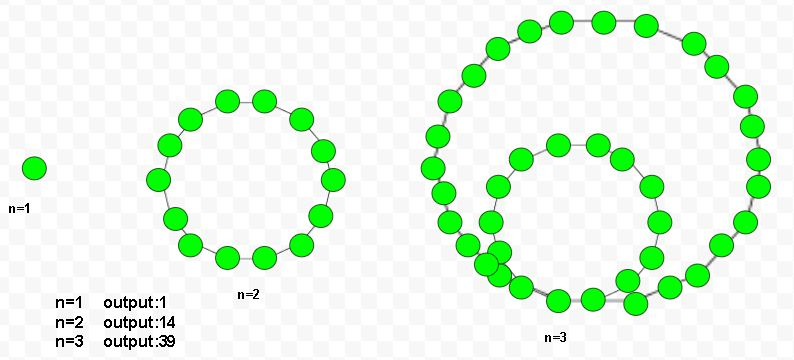• Last Updated : 20 May, 2022

Given a number n, the task is to find the nth tetradecagonal number. A tetradecagonal number is a 14-sided polygon called tetrakaidecagon or tetradecagon and belongs to the figurative number. The nth tetradecagonal number is dotted with some dots and create a series of pattern. They have a common sharing corner point and dotted with their spaces to each other. The dots continue with the nth nested loop.

Examples :

Input : 5
Output :125

Input :7
Output :259Formula for nth tetradecagonal number :-## C++

 // Program to find nth// Tetradecagonal number#include using namespace std; // Function to find// Tetradecagonal numberint tetradecagonal_num(int n){    // Formula to calculate nth    // tetradecagonal number=    return (12 * n * n - 10 * n) / 2;} // Driver Codeint main(){    int n = 2;    cout << n << " th Tetradecagonal number: ";    cout << tetradecagonal_num(n);    cout << endl;    n = 6;    cout << n << " th Tetradecagonal number: ";    cout << tetradecagonal_num(n);     return 0;}

## C

 // C Program to find nth// Tetradecagonal number#include  // Function to find// Tetradecagonal numberint tetradecagonal_num(int n){    // Formula to calculate nth    // tetradecagonal number=    return (12 * n * n - 10 * n) / 2;} // Driver Codeint main(){    int n = 2;    printf("%dth Tetradecagonal number: ",n);    printf("%d\n",tetradecagonal_num(n));     n = 6;    printf("%dth Tetradecagonal number: ",n);    printf("%d\n",tetradecagonal_num(n));     return 0;} // This code is contributed by kothavvsaakash.

## Java

 // Java Program to find nth// Tetradecagonal numberimport java.io.*; class GFG{     // Function to find// Tetradecagonal numberstatic int tetradecagonal_num(int n){    // Formula to calculate nth    // tetradecagonal number=    return (12 * n * n - 10 * n) / 2;} // Driver Codepublic static void main (String[] args){int n = 2;System.out.print(n + " th Tetradecagonal" +                              " number: ");System.out.println(tetradecagonal_num(n)); n = 6;System.out.print(n + " th Tetradecagonal" +                              " number: ");System.out.print(tetradecagonal_num(n)); }}// This code is code is// contributed by m_kit

## Python3

 # Program to find nth# Tetradecagonal number # Tetradecagonal number# number functiondef tetradecagonal_num(n) :         # Formula to calculate    # nth Tetradecagonal    # number return it    # into main function.    return (12 * n * n -            10 * n) // 2 # Driver Codeif __name__ == '__main__' :             n = 2    print(n,"th Tetradecagonal " +                     "number : " ,            tetradecagonal_num(n))     n = 6    print(n,"th Tetradecagonal " +                     "number : " ,            tetradecagonal_num(n)) # This code is contributed ajit

## C#

 // C# Program to find nth// Tetradecagonal numberusing System; class GFG{         // Function to find    // Tetradecagonal number    static int tetradecagonal_num(int n)    {             // Formula to calculate nth    // tetradecagonal number    return (12 * n * n -            10 * n) / 2;    } // Driver Codestatic public void Main (){    int n = 2;    Console.Write(n + "th Tetradecagonal" +                              " number: ");    Console.WriteLine(tetradecagonal_num(n));     n = 6;    Console.Write(n + "th Tetradecagonal" +                              " number: ");    Console.WriteLine(tetradecagonal_num(n));}} // This code is contributed by ajit

## PHP

 

## Javascript

 

Output :

2 th Tetradecagonal number: 14
6 th Tetradecagonal number: 186

Time Complexity: O(1)
Auxiliary Space: O(1)

My Personal Notes arrow_drop_up